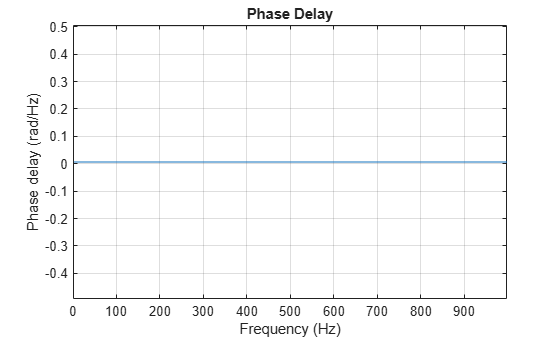# Group Delay and Phase Delay

The group delay of a filter is a measure of the average time delay of the filter as a function of frequency. The group delay is defined as the negative first derivative of the filter's phase response. If the complex frequency response of a filter is $H\left({e}^{j\omega }\right)$, then the group delay is

${\tau }_{\mathrm{g}}\left(\omega \right)=-\frac{\mathit{d}\theta \left(\omega \right)}{\mathit{d}\omega }$,

where $\theta \left(\omega \right)$ is the phase, or argument, of $H\left({e}^{j\omega }\right)$. Use the `grpdelay` function to compute group delay of a filter. For example, verify that, for a linear-phase FIR filter, the group delay is one-half the filter order.

```d = designfilt('lowpassfir','FilterOrder',20,'CutoffFrequency',200,'SampleRate',2000); islinphase(d)```
```ans = logical 1 ```
`grpdelay(d)`The phase delay of a filter is defined as the negative of the phase divided by the frequency:

${\tau }_{p}\left(\omega \right)=-\frac{\theta \left(\omega \right)}{\omega }$.

Use the `phasedelay` function to compute the phase delay of a filter. For the linear-phase FIR filter of the previous example, the phase delay is equal to the group delay.

`phasedelay(d)`Use FVTool to plot both the group delay and the phase delay of a system on the same graph. For example, plot the delays of a fifth-order Butterworth lowpass filter.

```d = designfilt('lowpassiir','FilterOrder',5,'HalfPowerFrequency',200,'SampleRate',2000); fvtool(d,'Analysis','grpdelay','OverlayedAnalysis','phasedelay','Legend','on')```